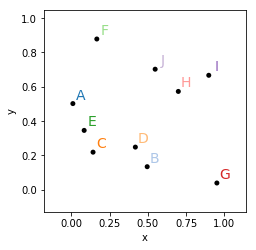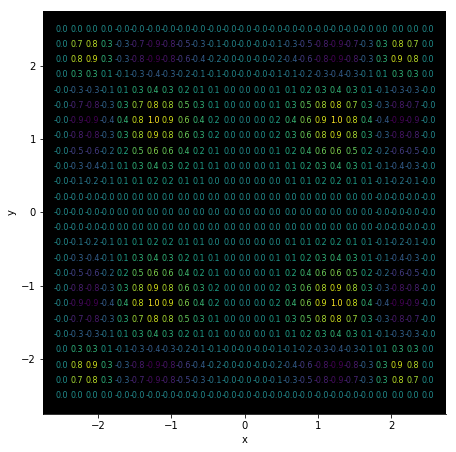# Labels¶

Title
Labels Element
Dependencies
Matplotlib
Backends
Matplotlib
Bokeh
In :
import numpy as np
import holoviews as hv
from holoviews import opts

hv.extension('matplotlib')The Labels element may be used to annotate a plot with a number of labels. Unlike the Text element, Labels is vectorized and allows plotting many labels at once. It also supports any tabular or gridded data format. This also means that most other elements may be cast to a Labels element to annotate or label the values.

Labels also support various options that make it convenient to use as an annotation, e.g. xoffset and yoffset options allow adjusting the position of the labels relative to an existing data point and the color option allows us to colormap the data by a certain dimension.

In :
np.random.seed(9)
data = np.random.rand(10, 2)
points = hv.Points(data)
labels = hv.Labels({('x', 'y'): data, 'text': [chr(65+i) for i in range(10)]}, ['x', 'y'], 'text')

(points* labels).opts(
opts.Labels(color='text', cmap='Category20', xoffset=0.05, yoffset=0.05, size=14, padding=0.2),
opts.Points(color='black', s=25))

Out:If the value dimension of the data is not already of string type it will be formatted using the applicable entry in Dimension.type_formatters or an explicit value_format defined on the Dimension. Additionally the color_index option allows us to colormap the text by a dimension.

Here we will create a 2D array of values, define a Dimension with a formatter and then colormap the text:

In :
value_dimension = hv.Dimension('Values', value_format=lambda x: '%.1f' % x)
xs = ys = np.linspace(-2.5, 2.5, 25)
zs = np.sin(xs**2)*np.sin(ys**2)[:, np.newaxis]

hv.Labels((xs, ys, zs), vdims=value_dimension).opts(
opts.Labels(bgcolor='black', cmap='viridis', color='Values', fig_size=200, padding=0.05, size=8))

Out: